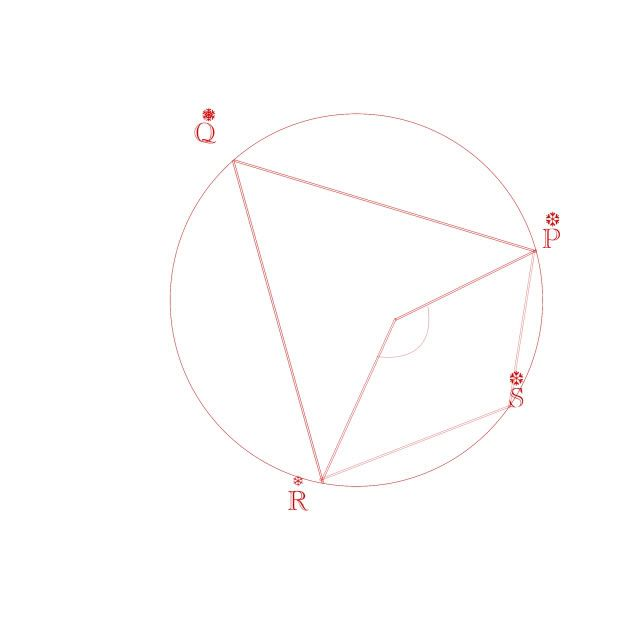Thread: Points on a circle

1. Points on a circle

In the diagram, take O as the centre of the circle.

P,q,R and S are points on the circle

Angle ROP=110

Calculate the angle RSP

I did 360-110
then 360-55
Then 2*(305-250)=110

rsp=1102. The correct way to do it is to make use of angle PQR.

From the properties of angles within a circle, we know that angle 2PQR = angle ROP

Therefore, angle PQR = 110/2 = 55

Now, from quadrilaterals inscribed within a circle, the sum of two opposite angles = 180 degrees.

Therefore, 180 - 55 = RSP

And RSP = 125 degrees

3. Interesting, do you have a place where I can shift through all of these laws? I'm studying for an exam and I have nothing but general knowledge to draw from, not knowing half of the requirements.

4.easiest and correct method

its very easy NO NEED TO CALCULATE ANGLE PQR
angle PSR=(1/2)(angle POR in the major segment)
angle POR in the major segment =360-110=250
therfor
angle PSR=(1/2)*250=125
this is the easiest and shortest method

5. Thanks but where do you get these methods? Point me towards a website, book, anything please

Search Tags

circle, points